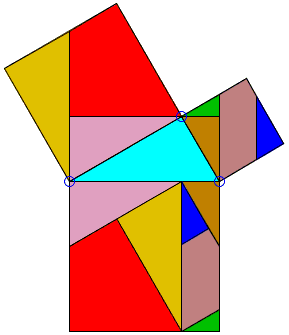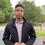# Invalid proofs of geometryWhy is this not a valid proof of the pythagorean theorem.Note by Mardokay Mosazghi
7 years, 3 months ago

This discussion board is a place to discuss our Daily Challenges and the math and science related to those challenges. Explanations are more than just a solution — they should explain the steps and thinking strategies that you used to obtain the solution. Comments should further the discussion of math and science.

When posting on Brilliant:

• Use the emojis to react to an explanation, whether you're congratulating a job well done , or just really confused .
• Ask specific questions about the challenge or the steps in somebody's explanation. Well-posed questions can add a lot to the discussion, but posting "I don't understand!" doesn't help anyone.
• Try to contribute something new to the discussion, whether it is an extension, generalization or other idea related to the challenge.
• Stay on topic — we're all here to learn more about math and science, not to hear about your favorite get-rich-quick scheme or current world events.

MarkdownAppears as
*italics* or _italics_ italics
**bold** or __bold__ bold
- bulleted- list
• bulleted
• list
1. numbered2. list
1. numbered
2. list
Note: you must add a full line of space before and after lists for them to show up correctly
paragraph 1paragraph 2

paragraph 1

paragraph 2

[example link](https://brilliant.org)example link
> This is a quote
This is a quote
    # I indented these lines
# 4 spaces, and now they show
# up as a code block.

print "hello world"
# I indented these lines
# 4 spaces, and now they show
# up as a code block.

print "hello world"
MathAppears as
Remember to wrap math in $$ ... $$ or $ ... $ to ensure proper formatting.
2 \times 3 $2 \times 3$
2^{34} $2^{34}$
a_{i-1} $a_{i-1}$
\frac{2}{3} $\frac{2}{3}$
\sqrt{2} $\sqrt{2}$
\sum_{i=1}^3 $\sum_{i=1}^3$
\sin \theta $\sin \theta$
\boxed{123} $\boxed{123}$

## Comments

Sort by:

Top Newest

Can it be generalized for all possible pairs of squares?

- 7 years, 3 months ago

Log in to reply

I give up. Can you explain why it is not a valid proof?

Staff - 7 years, 3 months ago

Log in to reply

Yeah, this is how I was taught in school actually (well, everyone already knew it, but the teacher thought it was a cool thing to help explain why it works).

Staff - 7 years, 3 months ago

Log in to reply

I am not sure, when I searched online I found this as the reason. While the proof looks like many other proofs by dissection and rearrangement, this one is not complete in that the construction does not go through where one of the legs of the given triangle is essentially less than the other This is the website:http://www.cut-the-knot.org/pythagoras/FalseProofs.shtml refer to proof #3

- 7 years, 3 months ago

Log in to reply

The main concern is that the picture doesn't deal with all possible cases. In this picture, we see that the small square is broken up into 3 vertical strips, and we can see how to rearrange them. The central strip juts nicely into the brown and blue triangles, with the green triangles placed below.

However, there is no reason why there are only 3 vertical strips. As the larger base grows larger, the smaller square could take many many vertical strips, after which it is unclear how to glue everything together. What is the role of the brown and blue triangles, and where do they go?

This is akin to drawing a special picture for a geometry question, say by setting the triangle to be equilateral or isosceles, and finding the answer for the specific case. We know that the answer is only valid in that scenario, and not in the more general case.

Staff - 7 years, 3 months ago

Log in to reply

Thanks

- 7 years, 3 months ago

Log in to reply

solve the theorem in the middle of square 3/5^2+4/5^2=25 make sq of 25=9+16=25

- 7 years, 1 month ago

Log in to reply

TRANGLE IN WHICH SIN=4/5^2=16/25 AND COS=3/5^2=9/25 SO EQUAL TO 25/25=1 THEREFORE 25=25 AFTER WHICH LEFT DISOLVE INTO 4^2+3^2=25 .IF ASSUME SIDE OF MIDDLE TRIANGLE ARE 3,4.,HYPOTINEOUS=5. PLEASE COMMENT.

- 7 years, 1 month ago

Log in to reply

all the sq are divided into right angle triangle. if the sq are of side are 3,4,5 , it will comes equal to the area of sq root 50 after measurement of each triangle . central triangle. figure cannot be drawn on same sheet ihave roof area equal to the three sq.

- 7 years ago

Log in to reply

my answer was 0.2 pleace comment differnce 0.217& 0.2

- 6 years, 10 months ago

Log in to reply

×

Problem Loading...

Note Loading...

Set Loading...